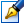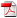##Topological algebraic systems and its applications

 Author: Liubomir Chiriac Degree: doctor habilitat of physics and mathematics Speciality: 01.01.04 - Geometry and topology Year: 2011 Scientific consultant: Mitrofan Ciobandoctor habilitat, professor Institution: Tiraspol State University Scientific council: DH 01-01.01.04-27.03.08 Institute of Mathematics and Computer Science of the ASM

### Status

The thesis was presented on the 19 April, 2011
Approved by NCAA on the 8 July, 2011

### Abstract0.45 Mb / in romanian

### Keywords

topological universal algebra, variety, topological quasigroup, multiple identities, omogen isotope, resoluble space, medial and paramedial grupoid, fuzzy algebra

### Summary

The thesis is written in English, contains introduction, 6 chapters, conclusions, 210 references, 200 pages of the basic text. The main results of the thesis are published in 45 scientific works. Keywords: topological universal algebra, variety, topological quasigroup, multiple identities, omogen isotope, resoluble space, medial and paramedial grupoid, fuzzy al- gebra.

The thesis is dedicated to the study: The in uence of the algebraic structures on the topological properties of the topological universal algebras. In particular, the topological algebraic systems and its applications in diverse fields is investigated. The purpose of the work resides in: mastering the studying methods of the topolo- gies on free algebras generated by pseudocompact and countable compact spaces; de- scribing the compact subsets of the free topological algebras and that of k-algebras; elaborating research methods regarding the topological quasigroups with multiple iden- tities; establishing a general theory on the decomposition of the topological groupoids with invertibility properties; solving the homomorphism problem for fuzzy algebras. Methodology of the research: the constructions and the methods of proofs are based on the notions of topological algebra, free algebra, variety, quasigroup with multiple identities, solvable space, fuzzy algebra.

The scientific innovation is determined by the solving of the following problems: there have been elaborated studying methods of topologies on free algebras gener- ated by pseudocompact and countable compact spaces; there have been determined the conditions for the continuous homomorphisms of the topological groupoids with continuous division to be open; there have been described the compact subsets of the free k-algebras; there have been established some topological properties, which are pre- served under the MK-equivalence relation; there have been introduced and analyzed the quasigroups with multiple identities; there has been elaborated a method of con- struction of the Haar measure on medial quasigroups; there has been elaborated a method of decomposition of special topological groupoids; there has been constructed a universal covering on topological E-algebras with continuous signature; are given a general solution of the homomorphism problem for fuzzy algebras.

The theoretical value of the work: there have been development of the general theories, elaborated the new concepts, methods and constructions which contributed to achieving goals and objectives of the research. The basic results of the work are new. The practical value: the methodology applied, the concepts and methods developed in work allowed to find the solution of concrete problems or some aspects of the prob- lems formulated by A.I Mal'cev, L.S. Pontrjagin, M.M Choban. Mathematical tools developed and applied led to solving problems in various areas of modern mathematics. Implementation: The results from this work can be used in the theory of topological universal algebras, of topological quasigroups, of automata, of fuzzy algebras, and in elaborating optional courses.

### Summary

1.BASIC CONSTRUCTIONS AND ANALYSIS OF THE CURRENT SITUATION IN TOPOLOGICAL ALGEBRA
• 1.1. Basic Topological Constructions
• 1.2. Basic Algebraical Constructions
• 1.3. On Methods of Constructing some Free Universal Algebras
• and Solving Equations over them…
• 1.4. The Basic Research Topic
• 1.5. Conclusions for Chapter 1

2. ON APPLYING UNIFORM STRUCTURES TO STUDY OF FREE TOPOLOGICAL ALGEBRAS
• 2.1. Mappings of Pseudocompact Spaces
• 2.2. Πω-Spaces
• 2.3. Cω-Spaces
• 2.4. Universal Algebras. Terms
• 2.5. Uniform Algebras
• 2.6. T-Uniform Algebras
• 2.7. T-Uniformization of Varieties.
• 2.8. Mapping Pseudocompact Sets in Free Algebras
• 2.9. Free Algebras of Πω-Spaces and Gω-Spaces
• 2.11. Free Algebras for P-Spaces
• 2.12. The Case of Topological Groups Rings, and Modules
• 2.13. Conclusions for Chapter 2.

3. TOPOLOGICAL GROUPOIDS AND QUASIGROUPS WITH MULTIPLE IDENTITIES
• 3.1. General Notes
• 3.2. Multiple Identities
• 3.3. Homogeneous Isotopes
• 3.4. The Homogeneous Isotopes and Congruencies
• 3.5. General Properties of Medial Quasigroups
• 3.6. On Haar Measures on Medial Quasigroups
• 3.7. Examples of Quasigroups with Multiple Identities
• 3.8. On Medial and Paramedial Topological Groupoids
• 3.9. Some Properties of (n,m)-homogeneous Isotopies
• 3.10. Paramedial Topological Groupoids
• 3.11. On Subquasigroup of the Topological Quasigroup
• 3.12. Embedding Topological Groupoids
• 3.13. On Homomorphisms of Abstract and Semitopological Quasigroups
• 3.14. Homomorphism of topological groupoids with the continuous division
• 3.15. On The Medial Quasigroups
• 3.16. Covering Algebras. Preservation Properties in the Locally Trivial Fibering
• 3.17. Universal Covering Algebras
• 3.18. Examples of Covering Algebras .
• 3.19. Conclusions for Chapter 3

4. COMPACT SUBSETS OF FREE ALGEBRAS WITH TOPOLOGIES AND EQUIVALENCE OF SPACES
• 4.0.1 Notations and remarks
• 4.1. Spaces and mappings
• 4.2. Algebras with Topologies
• 4.3. Free Algebras of μ-Complete Spaces
• 4.4. On M-equivalence of spaces
• 4.5. One Special Construction
• 4.6. Homotopy Classes of Mappings
• 4.7. Homotopy Classes of Homomorphisms
• 4.8. On Homotopical Cohomology
• 4.9. Spaces of Mappings
• 4.10. Conclusions for Chapter 4

5. RESOLVABILITY OF SOME SPECIAL ALGEBRAS WITH TOPOLOGIES
• 5.1. Introductory Notions
• 5.2. Groupoids with Invertibility Properties
• 5.3. Topologies on Algebras
• 5.4. Decomposition of InPk-n-groupoids
• 5.5. Decomposition of InP-n-groupoids
• 5.6. Conclusions for Chapter 5

6. ON FUZZY ALGEBRAS
• 6.1. The Lattice of L-fuzzy Algebras
• 6.2. The Fuzzy Homomorphisms
• 6.3. Case of the Proper Homomorphisms
• 6.4. Case of Distributive Lattices
• 6.5. Case of Dense Homomorphism
• 6.6. Algebras with Fuzzy Operations
• 6.7. On Fuzzy Finitely Generated Qroupoids
• 6.8. The Basis of the Fuzzy Algebras
• 6.9. Conclusions for Chapter 6